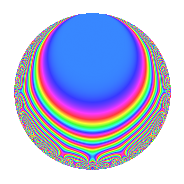# Properties

 Label 36.2.hLevel 36 Weight 2 Character orbit h Rep. character $$\chi_{36}(11,\cdot)$$ Character field $$\Q(\zeta_{6})$$ Dimension 8 Newforms 1 Sturm bound 12 Trace bound 0

# Related objects

## Defining parameters

 Level: $$N$$ = $$36 = 2^{2} \cdot 3^{2}$$ Weight: $$k$$ = $$2$$ Character orbit: $$[\chi]$$ = 36.h (of order $$6$$ and degree $$2$$) Character conductor: $$\operatorname{cond}(\chi)$$ = $$36$$ Character field: $$\Q(\zeta_{6})$$ Newforms: $$1$$ Sturm bound: $$12$$ Trace bound: $$0$$

## Dimensions

The following table gives the dimensions of various subspaces of $$M_{2}(36, [\chi])$$.

Total New Old
Modular forms 16 16 0
Cusp forms 8 8 0
Eisenstein series 8 8 0

## Trace form

 $$8q - 3q^{2} - q^{4} - 6q^{5} - 3q^{6} - 6q^{9} + O(q^{10})$$ $$8q - 3q^{2} - q^{4} - 6q^{5} - 3q^{6} - 6q^{9} - 8q^{10} + 6q^{12} - 2q^{13} + 12q^{14} - q^{16} + 18q^{18} + 18q^{20} - 6q^{21} + 3q^{22} + 3q^{24} - 6q^{25} - 12q^{28} + 6q^{29} - 18q^{30} - 33q^{32} + 24q^{33} + 7q^{34} - 33q^{36} - 8q^{37} - 27q^{38} + 10q^{40} + 24q^{41} - 18q^{42} + 6q^{45} + 12q^{46} + 21q^{48} - 10q^{49} + 21q^{50} + 16q^{52} + 39q^{54} + 18q^{56} + 6q^{57} + 4q^{58} + 6q^{60} - 2q^{61} + 26q^{64} - 30q^{65} - 24q^{66} - 15q^{68} - 30q^{69} - 6q^{70} - 21q^{72} + 4q^{73} - 30q^{74} - 3q^{76} - 30q^{77} - 12q^{78} - 30q^{81} + 10q^{82} + 30q^{84} + 8q^{85} + 21q^{86} - 21q^{88} + 6q^{90} + 24q^{92} + 30q^{93} - 18q^{94} + 12q^{96} + 4q^{97} + O(q^{100})$$

## Decomposition of $$S_{2}^{\mathrm{new}}(36, [\chi])$$ into irreducible Hecke orbits

Label Dim. $$A$$ Field CM Traces $q$-expansion
$$a_2$$ $$a_3$$ $$a_5$$ $$a_7$$
36.2.h.a $$8$$ $$0.287$$ 8.0.170772624.1 None $$-3$$ $$0$$ $$-6$$ $$0$$ $$q+(1-\beta _{1}+\beta _{4}+\beta _{7})q^{2}+(-\beta _{2}-\beta _{3}+\cdots)q^{3}+\cdots$$